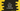# C++ program to convert binary to decimal## C++ program to convert binary to decimal:

Binary number system is a base-2 numeral system. Numbers represented in binary number system are called binary numbers. All numbers in binary number system are represented by 0 and 1.

Decimal number system is base-10 numeral system and we have digits 0 to 9 to represent numbers.

In this post, we will learn how to convert a binary value to its decimal representation.

### How to convert a binary value to decimal:

The formula to convert a binary to decimal is easy. We need to find the sum of all multiplications of binary digits and power of 2 at that position to find the decimal value for a binary.

For example, if abcde is a binary number with a, b, c, d, e are its digits. start from right to left. The position of the rightmost element is 0 and increment 1 for each to its left.

So, its decimal value will be:

``e * 2^0 + d * 2 ^ 1 + c * 2^2 + b * 2^3 + a * 2^4``

## C++ program to convert binary to decimal:

Below program converts a binary number to decimal:

``````#include <iostream>

using namespace std;

int binaryToDecimal(int binary)
{
int decimal = 0;
int multiplier = 1;

while (binary)
{
int lastDigit = binary % 10;
binary = binary / 10;

decimal += multiplier * lastDigit;
multiplier *= 2;
}

return decimal;
}
int main()
{
int binary = 101001;

cout << "Binary: " << binary << ", Decimal " << binaryToDecimal(binary) << endl;

return 0;
}``````

Here,

• binaryToDecimal method takes the binary value as the parameter and converts it to decimal and returns that.

• It uses a while loop to calculate the decimal result.
• The loop finds the last digit of the binary number and multiplies it with the multiplier i.e. 2^n. It is initialized as 1 since 2^0 is 1. On each iteration, we are multiplying this value by 2.
• Inside the loop, it updates the decimal value by adding multiplier * lastDigit.
• Also, the last digit of the binary number is removed on each step.
• Once the while loop ends, it will return the decimal value.

If you run the above program, it will print the below output:

``Binary: 101001, Decimal 41``

## C++ program to convert binary to decimal by using inbuilt function:

C++ STL provides a method called stoi() to convert a string to integer. We can use this method to convert a string to numbers in different formats like binary, octal, hexadecimal etc.

Below program uses stoi to convert a binary string to decimal:

``````#include <iostream>
#include <string>

using namespace std;

int main()
{
string binary = "101001";
int decimal = stoi(binary, nullptr, 2);

cout << "Binary: " << binary << ", Decimal " << decimal << endl;

return 0;
}``````

It will give a similar result. The third argument is 2, which defines that we are converting the string from binary.

Below is the output it will print: